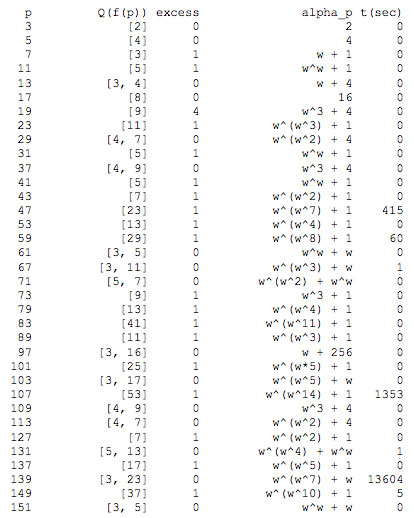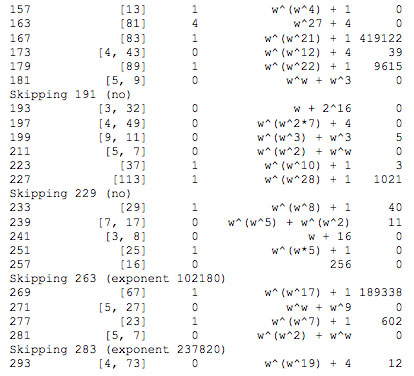One of the coolest (pure math) facts in Conway’s book ONAG is the explicit construction of the algebraic closure $\overline{\mathbb{F}_2}$ of the field with two elements as the set of all ordinal numbers smaller than $(\omega^{\omega})^{\omega}$ equipped with nimber addition and multiplication.

Some time ago we did run a couple of posts on this. In transfinite number hacking we recalled Cantor’s ordinal arithmetic and in Conway’s nim arithmetics we showed that Conway’s simplicity rules for addition and multiplication turns the set of all ordinal numbers into a field of characteristic zero : $\mathbb{On}_2$ (pronounced ‘Onto’).

In the post extending Lenstra’s list we gave Hendrik Lenstra’s effective construction of the mystery elements $\alpha_p$ (for prime numbers $p$) needed to do actual calculations in $\mathbb{On}_2$. We used SAGE to check the values for $p \leq 41$ and solved the conjecture left in Lenstra’s paper Nim multiplication that $(\omega^{\omega^{13}})^{43} = \omega^{\omega^7} + 1$ and determined $\alpha_p$ for $p \leq 67$.Aaron Siegel has now dramatically extended this and calculated the $\alpha_p$ for all primes $p \leq 181$. He mails :

“thinking about the problem I figured it shouldn’t be too hard to write a dedicated program for it. So I threw together some Java code and… pushed the table up to p = 181! You can see the results below. Q(f(p)), excess, and alpha_p are all as defined by Lenstra. The “t(sec)” column is the number of seconds the calculation took, on my 3.4GHz iMac. The most difficult case, by far, was p = 167, which took about five days.

I’m including results for all p < 300, except for p = 191, 229, 263, and 283. p = 263 and 283 are omitted because they involve computations in truly enormous finite fields (exponent 102180 for p = 263, and 237820 for p = 283). I'm confident that if I let my computer grind away at them for long enough, we'd get an answer... but it would take several months of CPU time at least. p = 191 and 229 are more troubling cases. Consider p = 191: it's the first prime p such that p-1 has a factor with excess > 1. (190 = 2 x 5 x 19, and alpha_19 has excess 4.) This seems to have a significant effect on the excess of alpha_191. I’ve tried it for every excess up to m = 274, and for all powers of 2 up to m = 2^32. No luck.”

Aaron is writing a book on combinatorial game theory (to be published in the AMS GSM series, hopefully later this year) and will include details of these computations. For the impatient, here’s his listPublished in math number theory